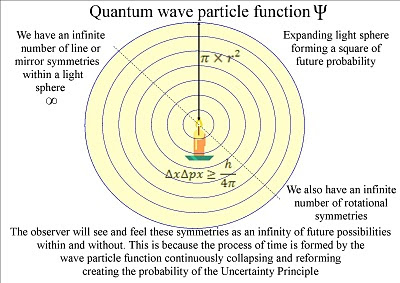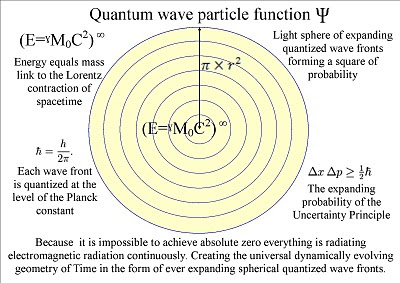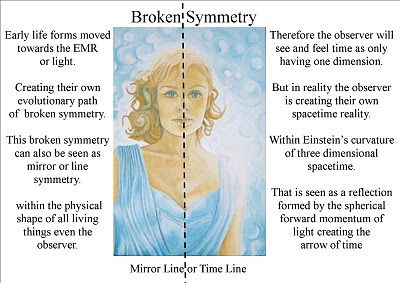Tuesday, 13 April 2010

Is time the Hidden Variable of Quantum Physics?

A new theory on the paradoxes of Quantum Physics

(Quantum Atom Theory)
~

Quantum Atom Theory was formed out of the very simple idea that the probability of Heisenberg Uncertainty Principle of quantum physics is the same probability that the observer will have with any future event.

This makes time the Hidden Variable of quantum physics. We know that time is a variable because we have time dilation when an object accelerates towards the speed of light and around objects of great mass.

A Hidden variable would have to affect the whole Universe and the dynamics of time is the only variable that has done just that. Time is a measurement and a variable and this is why we have the Measurement Problem in quantum physics.

Light cannot be a wave and a particle (photon) at the same time. When something seems to be both true and false at the same time it means that our reasoning must be wrong or that our concept of time is wrong.

The dynamics of light and time are linked together light moves in straight lines but in three dimensional space it will expand out in all directions forming light spheres.

The paradoxes of Quantum Physics can be explained by simple geometry each light sphere will create a volume of space that will have a square of probability.

The probability of when and where the waves of light will come in contact with an electron forming an electron photon coupling creating a new photon of energy.

Therefore each new photon of energy will have a unique position in space and time that will only be relative to the wavelength of the light.

In this way the forward momentum of EMR or light creates the time continuum that the observer will see and feel as the passage of time.

Time has the geometry of spacetime that is formed by the continuous emission and absorption of electromagnetic radiation or light from one Atom to another.

Because it is impossible to achieve absolute zero all atoms radiate EMR continuously creating a continuous process of symmetry forming and breaking this gave the evolution of life a mathematical base.

The atoms will bond together forming their own spacetime geometry even the atoms of the observer have created their own spacetime geometry. Therefore Heisenberg Uncertainty Principle is the same uncertainty that the observer will have with any future event the uncertainty of everyday life.

The polarization of the light will be the same for the entire surface of the light sphere therefore the photon will have opposite spin on opposite sides of the light sphere creating quantum entanglement and the symmetry and geometry of spacetime.

Because this is a continuous process at the same rate that light moves the speed of light between the atoms will always be a universal constant independent of the motion of the source.

Quantum Atom Theory is an interpretation of quantum physics that gives us an explanation for the mathematical and physical shape of the real world.

~

Link to Quantum Atom Theory on You Tube

~

Friday, 2 April 2010

The symmetry of Light and Geometry of Time in Quantum Physics

The Symmetry of Light and Geometry of Time within Quantum Physics
~Out of very simple geometry and mathematics diversity will form into complexity

~Quantum Atom Theory is an interpretation of quantum physics that gives us an explanation for the mathematics and physical shape of the real world.
~Newton said every action will create an opposite reaction, in Quantum Atom Theory the inward force of gravity is the opposite reaction to the outward momentum of EMR.

~Energy or mass will form their own spacetime geometry that will be visible in mathematics and in the physical shape of all living things.

~The observer will create their own broken symmetry and will therefore be at the centre of their own reflection found.

~

Link to Quantum Atom Theory on You Tube

~